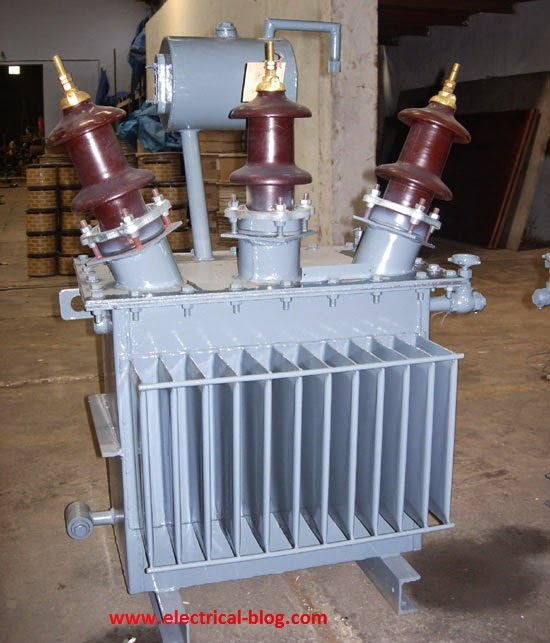# Three Phase Transformers BasicsA three-phase transformer is used to generate and transmit a large amount of power. Three-phase step up and step down transformers are basically used in various fields in the power system network. A transformer can be formed by joining three separate single-phase winding in a suitable way. Such an arrangement is called 3-phase bank of the transformer. Otherwise, a single 3 phase transformer is one where the cores and winding of all three phases are combined in a single structure.

#### Advantage of a three-phase unit transformer

• A three-phase unit transformer has the following advantages over the 3 single-phase transformer of same KVA rating such as,
• It takes less space
• It is lighter, smaller and cheaper compared to the other one.
• Only three terminals are needed rather than six terminals in a single 3 phase transformer.
• The installation cost for the three-phase transformer is much lower than the other one.

#### Advantage of a transformer bank or three single-phase transformer

• A transformer bank or three single-phase transformer has the following advantages over a 3 phase transformer of same KVA rating such as,
• A transformer bank may be provided with higher KVA rating to supply an imbalanced load.
• When a transformer is damaged in a transformer bank then remaining two transformers may be used as open Δ (delta) connection.
• Transformer tank is less costly compared to a single-phase transformer.
• The transportation of a single-phase transformer is more convenient

But generally, we use a three-phase transformer rather than a transformer tank.

#### Construction of three-phase transformer

Construction of the magnetic core of a three-phase transformer may be understood by considering three single-phase transformer positioned at 1200  to each other. If three-phase sinusoidal voltage is applied to the winding then the flux produced φR, φS, φT will also be sinusoidal and balanced. If the three legs of the transformer carrying these fluxes are merged, the total flux in the merged leg will be zero.so these legs can be removed as it carries no flux. Usually, the structure is not convenient to build so the structure with the tree limbs in the same plane is used. Here the constructions of core transformer are shown in the figure.

Here the constructions of core transformer are shown in the figure.

#### Conditions of Three-Phase transformer in Parallel

• There are some conditions which must be fulfilled before connecting two 3-phase transformers in parallel. These are
• The polarities of the transformers must be the same.
• The primary and secondary voltage rating should be identical.
• Impedance is inversely proportional to the KVA ratings.
• Identical X/R ratio in the transformer impedance.
• The phase sequence of transformers must be the same.
• The phase shift between primary and secondary voltage must be the same for all the transformers.